# ACT Math - Exponents: Help and Review Chapter Exam

Exam Instructions:

Choose your answers to the questions and click 'Next' to see the next set of questions. You can skip questions if you would like and come back to them later with the yellow "Go To First Skipped Question" button. When you have completed the practice exam, a green submit button will appear. Click it to see your results. Good luck!

### Page 1

#### Question 1 1. Simplify using ONLY positive exponents.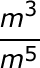#### Question 2 2. Simplify the expression using positive exponents.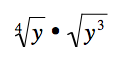#### Question 3 3. Simplify using only positive exponents.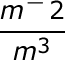#### Question 4 4. Which of the following is equivalent to the following expression?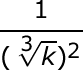#### Question 5 5. Simplify this expression using positive exponents.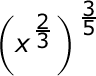### Page 2

#### Question 6 6. Which of the following is equivalent to the expression below?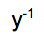#### Question 7 7. Which of the following radical expressions is equal to this exponential expression?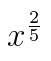#### Question 8 8. Simplify the following expression using positive exponents only.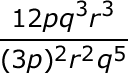#### Question 9 9. Simplify the following expression.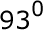#### Question 10 10. Which of the following is equivalent to the expression below?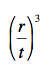### Page 3

#### Question 11 11. Simplify the following expression.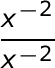#### Question 12 12. Which of the following would be true regarding the following exponential expression?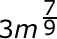#### Question 13 13. Which of the following is equivalent to the following expression?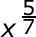#### Question 14 14. Simplify the expression below using only positive exponents.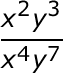#### Question 15 15. Simplify using ONLY positive exponents.### Page 4

#### Question 16 16. Simplify the following expression using positive exponents.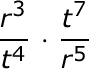#### Question 17 17. Simplify the expression below.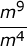#### Question 18 18. Which of the following exponential expressions is equal to this radical expression?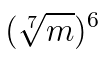#### Question 19 19. Which of the following is equivalent to the expression below?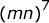#### Question 20 20. Simplify the expression below using positive exponents.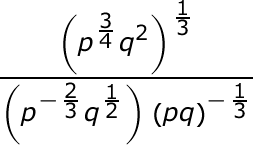### Page 5

#### Question 22 22. Using positive exponents, simplify the expression.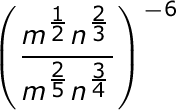#### Question 23 23. Which of the following is equivalent to the expression below?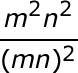#### Question 24 24. Simplify the following expression using positive exponents.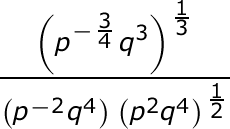#### Question 25 25. Simplify the expression below using positive exponents only.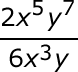#### ACT Math - Exponents: Help and Review Chapter Exam Instructions

Choose your answers to the questions and click 'Next' to see the next set of questions. You can skip questions if you would like and come back to them later with the yellow "Go To First Skipped Question" button. When you have completed the practice exam, a green submit button will appear. Click it to see your results. Good luck!

Support